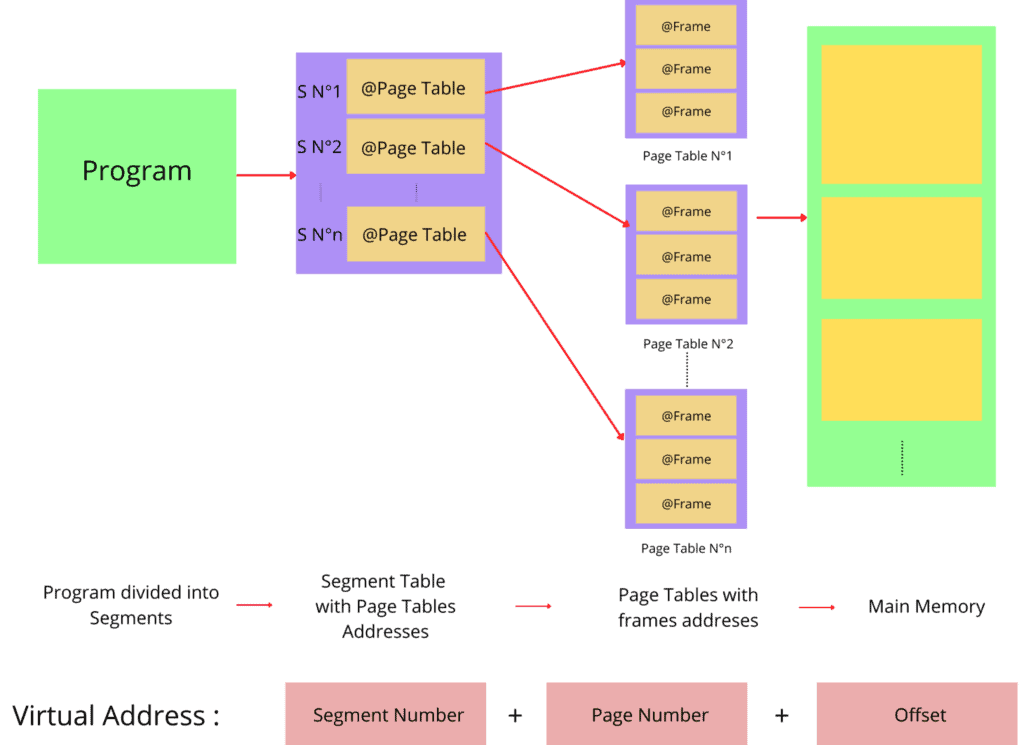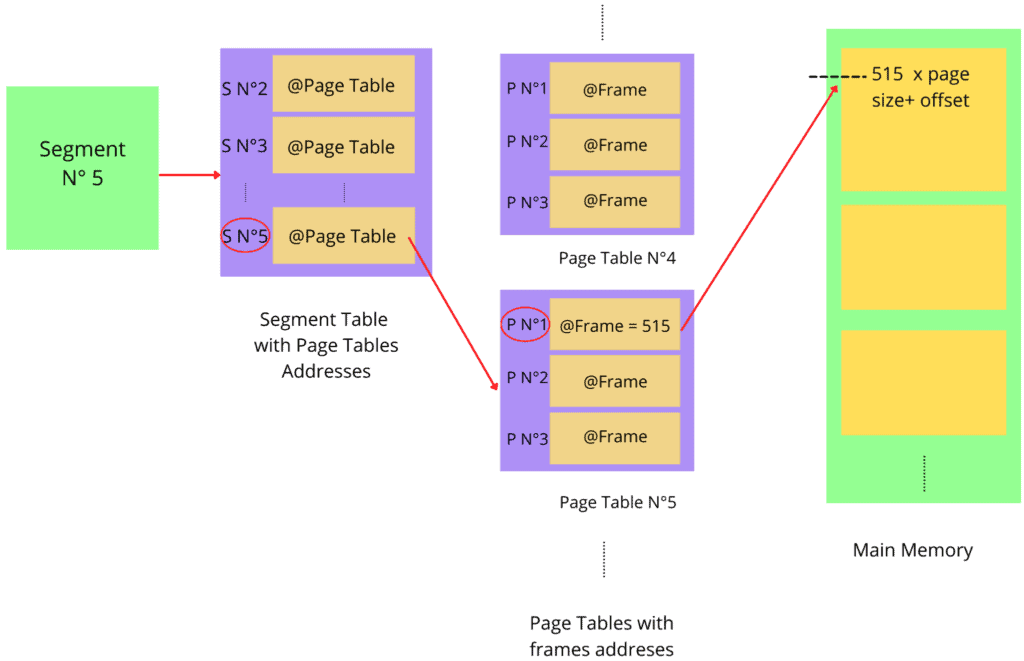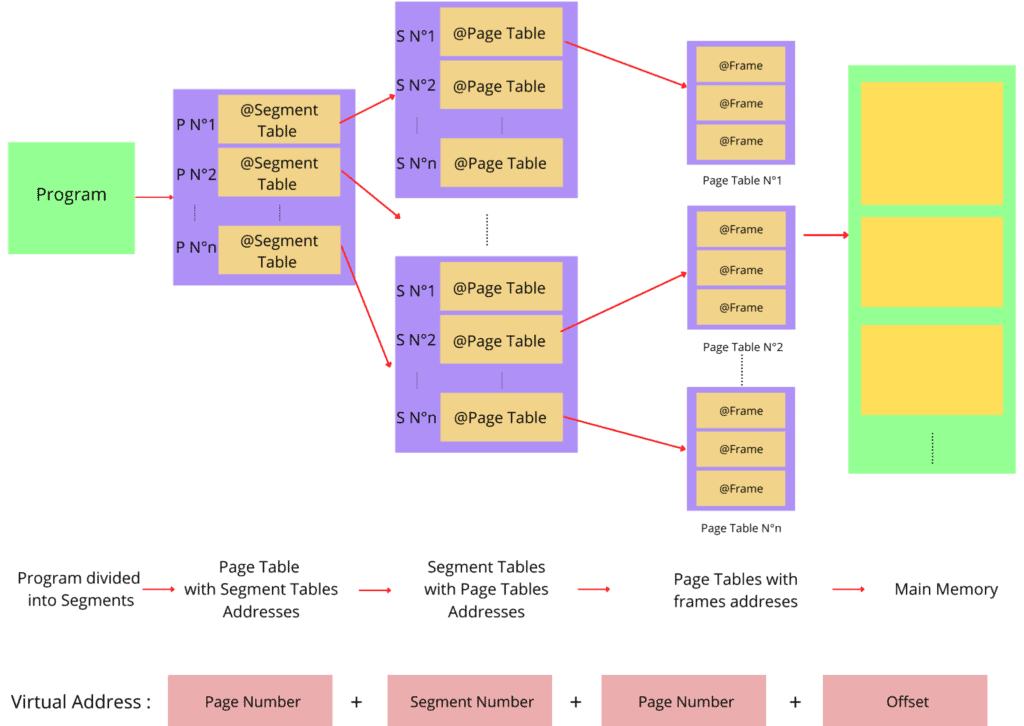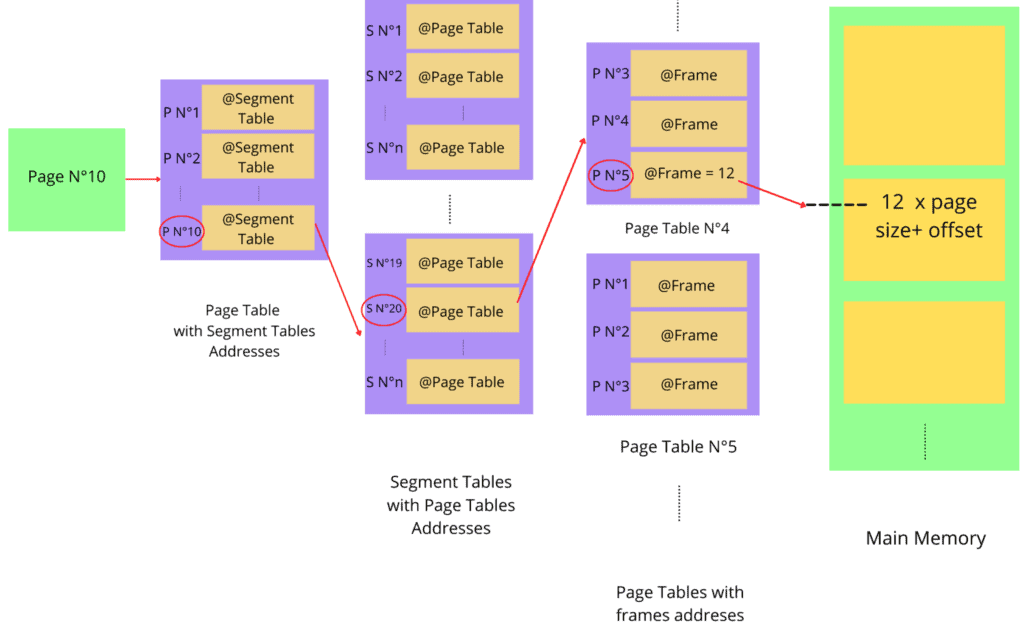## 1. Overview

In this tutorial, we’ll discuss the two most popular non-contiguous memory allocation techniques: segmented paging and paged segmentation. We’ll explore the way both methods work, their advantages and disadvantages.

Finally, we’ll present the core difference between them.

## 2. Motivation

The optimal use of a computer’s memory capacity has been the subject of extensive studies. With the advancement of computers, programs are also getting more complex and require more memory for execution.

In any machine, we need to share memory between multiple programs. However, it can cause the processing to be slow as the CPU needs to load data into RAM for each one of them.

We can’t determine the limit of how many programs can be executed on a computer. Hence, the size of the RAM would never be enough for a modern computer.

Now to solve the memory issue, one solution is to store the data in secondary memory. Then, when needed, a computer can retrieve data from the secondary memory to RAM. The operating system also uses virtual memory to tackle the physical shortage of memory and allot a temporary memory to a computer.

The problem with this approach is the page table may occupy a lot of memory in RAM if the logical address space is big. Hence, we need memory management techniques that can reduce the page table’s size in RAM.

## 3. Introduction to Segmented Paging

To reduce the size of the page table in RAM, we use a strategy that combines both segmentation and paging. But before going into the details of the combined strategy, first, let’s discuss the problems with paging.

Paging allows jobs and processes to be stored as a discontinuous space in memory. Thus, it solves the problem of external fragmentation.

To implement this technique, we divide the processes into fixed-size blocks. These blocks are called pages. We also divide physical memory into fixed-size blocks called frames.

When the OS executes a process, the CPU converts each page’s logical address to a physical address. Furthermore, the address generated to access the frame is divided into a page number and a frame number.

The main limit of the paging technique resides within the fact that when the virtual address is large, pages will take a large space in actual memory. In general, programs tend to be large. Therefore, they would consume a considerable amount of memory. As a result, some addresses would be invalid.

To solve this problem, we can use segmentation along with paging in order to reduce page table size. Generally, we can divide a program into four segments: the code segment, data segment, stack segment, and heap segment.

Ideally, there is no limit to the number of segments a process can be divided into. After splitting a process into segments, we create a segment table for each of them. Next, we divide each segment into fixed-size pages.

Thus, each segment entry in the segment table points to the page table that stores the frame addresses corresponding to the segment. This process considerably reduces the size of the page table.

When we use the segmented paging technique, we partition the address provided by the CPU into segment number, page number, and page offset.

Now to convert the logical address to a reel address, we need the frame addresses. We obtain the frame addresses from the segment table. Finally, the page table points to the frames of the segment in the main memory:Let’s summarize the whole process. At first, we divide the programs into segments. Each segment contains a segment table. Each segment table stores the addresses of the page tables. Page tables contain the frame address, which points to the main memory.

### 3.2. Example

Let’s take as an example in order to understand the practical use of the segmented paging technique. We’re taking a program with a function and the data it uses.

We divide the program into two segments: (A) for the function and (B) for the data. In the segment table, there’ll be cases for segments (A) and (B).

If the virtual address of (A) is:The physical address will be.

The main advantage of segmented paging is the memory usage reduction. Since it allocates fixed-size pages, it doesn’t cause external fragmentation. It makes memory allocations simpler.

The main drawback is external fragmentation. However, the probability of external fragmentation occurring is significantly less. The segmented paging technique also requires more hardware resources. The translation is sequential, which increases the memory access time, leading to higher complexity. Page tables have to be contiguous to be stored in memory.

## 4. Introduction to Paged Segmentation

In segmentation, we divide each process into segments. Each segment has a different size. Segments are loaded in the logical address memory space, which is a set of segments with various lengths.

Each segment has a name and a length. To execute a segment, its logical address is loaded to the physical memory space.

Generally, we refer to a segment by its number instead of name. Hence, to access a segment, its number and offset are required. We use the number as an index in the segment table mapped by the operating system. The value of the offset helps to determine the limit of the segment.

Joined together, the segment number and the offset value generate the segment address in physical memory space.

Nevertheless, segmentation presents many disadvantages. It allocates blocks of variable size in physical memory. Hence, segmentation can cause external fragmentation.

Paged segmentation was proposed as a solution to improve memory management. The paged segmentation technique consists of partitioning the segment table into pages, reducing the size of the segments table. Here, we divide the segments into pages.

In paged segmentation, firstly, we divide the segment table into pages. Therefore, we create a page table. Consequently, the virtual address consists of four fields: the page number, segment number, second-page number, and offset:Let’s summarize the whole process. At first, we divide the programs into segments. There exists a segment table for each segment. Then, we also create page tables that also contain the segment table addresses. In the page table, we can find the frame addresses which point to the main memory.

### 4.2. Example

Let’s take as an example in order to understand the paged segmentation technique practically. We’re taking a program withsegments (A), (B), and (C).

Let’s assume the virtual address of segment (C) is equal to. We need to access its physical address to extract some information.

Firstly, we need to know the corresponding segment table address, which is on thepage of the page table. Next, we require to extract the page table address corresponding to the segment from theentry of the previous page table. Finally, the frame address can be found at thepage of the last page table and added to the offset:The physical address will be.

The main advantage of paged segmentation is eliminating external fragmentation and reducing the page table size.

On the other hand, the problem of internal fragmentation is still not solved completely. There are occasions where internal fragmentation can occur, but the probability is less. There exist delays when we access the memory. Also, the hardware required for the implementation of paged segmentation technique is complex.

## 5. Differences

Let’s look at the main differences between paged segmentation and segmented paging:## 6. Conclusion

Despite some advantages of paging and segmentation, they remain one of the leading causes of memory fragmentation. To reduce the chances of memory fragmentation, combined memory management techniques are developed.

In this tutorial, we discuss two memory allocation techniques: segmented paging and paged segmentation. We presented the basic concept of each technique with an example demonstrating the work procedure.

Finally, we talked about the core differences between them.